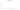ر.ع.‏

Omani Rial

how has the value of the currency changed in the last year?USD 1 = OMR 0.38499

How much is10 US dollars worth inOmani rials?
At the current exchange rate, 10 US dollars is worth 3.85 Omani rials
How much is50 US dollars worth inOmani rials?
At the current exchange rate, 50 US dollars is worth 19.25 Omani rials
How much is100 US dollars worth inOmani rials?
At the current exchange rate, 100 US dollars is worth 38.5 Omani rials
How much is500 US dollars worth inOmani rials?
At the current exchange rate, 500 US dollars is worth 192.5 Omani rials
How much is2,000 US dollars worth inOmani rials?
At the current exchange rate, 2,000 US dollars is worth 769.98 Omani rials
How much is10 US dollars worth inOmani rials?
At the current exchange rate, 10 US dollars is worth 3.85 Omani rials
How much is50 US dollars worth inOmani rials?
At the current exchange rate, 50 US dollars is worth 19.25 Omani rials
How much is100 US dollars worth inOmani rials?
At the current exchange rate, 100 US dollars is worth 38.5 Omani rials
How much is500 US dollars worth inOmani rials?
At the current exchange rate, 500 US dollars is worth 192.5 Omani rials
How much is2,000 US dollars worth inOmani rials?
At the current exchange rate, 2,000 US dollars is worth 769.98 Omani rials